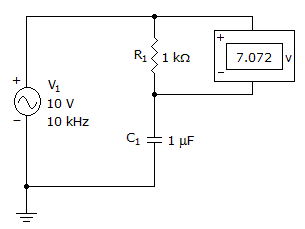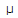# Electronics - Capacitors

### Exercise :: Capacitors - General Questions

61.

The strength of an electric field at a given point is proportional to the potential difference on the plates and inversely proportional to the:

 A. plate separation B. negative plate only C. field strength D. charge difference

Explanation:

No answer description available for this question. Let us discuss.

62.

How is ac current related to ac voltage in a purely capacitive circuit?

 A. AC current is 0.707 of the ac voltage. B. AC current lags ac voltage. C. AC current is 0.637 of the ac voltage. D. AC current leads ac voltage.

Explanation:

No answer description available for this question. Let us discuss.

63.

What is the voltage across the capacitor?A. 7.07 V B. 1.11 V C. 111 mV D. 7.07 mV

Explanation:

No answer description available for this question. Let us discuss.

64.

For a given charge difference, electrostatic field strength varies according to:

 A. plate differential B. plate area C. plate distance D. dielectric quantity

Explanation:

No answer description available for this question. Let us discuss.

65.

A capacitor has 25 V across its plates and a stored charge of 1500C. What is the capacitance value?

 A. 60 pF B. 60F C. 16.67 mF D. 37.5 mF